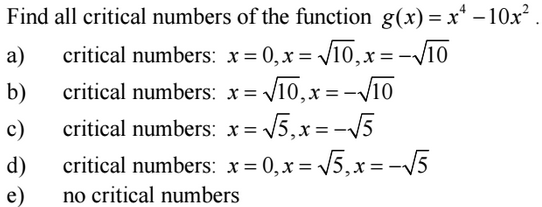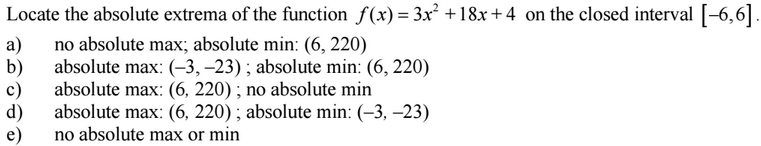### Day 39 - Rolle's Theorem and Mean Value Theorem - 03.09.15

Update
• Summative Exam 1
• Average = 80%
• Unit 3 Test on 3/27!
• If you are leaving for spring break early, please make arrangements with me to take the test before break!
• Last Week to Retake Unit 2 Test
• Parent-Teacher Conferences will be on Friday!
• Questions on previous Checkpoints?

Questions

Bell Ringer
1. Critical Numbers1. ExtremaReview
• How is the derivative used to locate the minimum and maximum values of a function on a closed interval?
• Find critical numbers using differentiation.
• Find extrema on a closed interval using differentiation.

Lesson
• Rolle's Theorem and Mean Value Theorem

Exit Ticket
• N/A
Lesson Objective(s)
• How are Rolle's Theorem and Mean Value Theorem related to differentiation?
Skills
1. Apply understanding of Rolle’s Theorem and the Mean Value Theorem.

#### In-Class Help Requests

Standard(s)
• APC.4
• Investigate asymptotic and unbounded behavior in functions.
• Includes:
• describing and understanding asymptotes in terms of graphical behavior and limits involving infinity
• APC.8
• Apply the derivative to solve problems.
• Includes:
• ​analysis of curves and the ideas of concavity and monotonicity
• optimization involving global and local extrema

Past Checkpoints